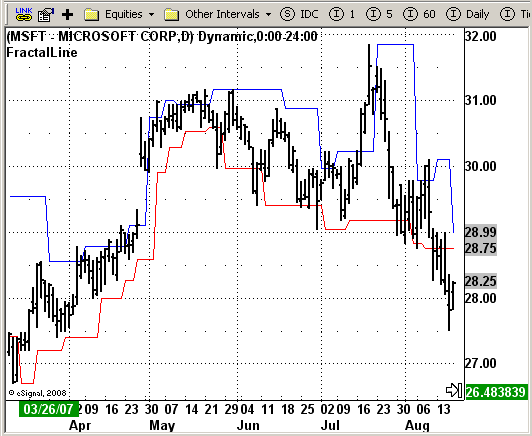# Bill Williams FractalLine

ICE Data Services -

 ABillW_FractalLine.efs EFSLibrary - Discussion Board

File Name: ABillW_FractalLine.efs

Description:
Bill Williams. FractalLine

Formula Parameters:
Strength: 2

Notes:
According to Bill Williams, one should enter the market after the new price
top (for long positions) or bottom (for short positions) has been broken
through. The identification here is based on defining the genuine fractal
formation; an upward fractal is detected if there is a row (at least three)
increasing values and after the local top has been achieved, bars (at least
two bars) show a constant descent. So the model requires at least 5 bars of
which the middle one is the local top (upward fractal) or the local bottom
(downward fractal) Entry orders according to this concept are usually posted
as stop orders activated after the price breaks through a preset level, where
the identification of the market`s leaving the previous price corridor takes place.
This indicator displays such levels, relative to which one decided whether the
price has broken out of the previous corridor.`/*********************************Provided By: eSignal (Copyright c eSignal), a division of Interactive Data Corporation. 2008. All rights reserved. This sample eSignal Formula Script (EFS) is for educational purposes only and may be modified and saved under a new file name. eSignal is not responsible for the functionality once modified. eSignal reserves the right to modify and overwrite this EFS file with each new release.Description: Bill Williams. FractalLineVersion: 1.0 10/14/2008Notes: According to Bill Williams, one should enter the market after the new price top (for long positions) or bottom (for short positions) has been broken through. The identification here is based on defining the genuine fractal formation; an upward fractal is detected if there is a row (at least three) increasing values and after the local top has been achieved, bars (at least two bars) show a constant descent. So the model requires at least 5 bars of which the middle one is the local top (upward fractal) or the local bottom (downward fractal) Entry orders according to this concept are usually posted as stop orders activated after the price breaks through a preset level, where the identification of the market`s leaving the previous price corridor takes place. This indicator displays such levels, relative to which one decided whether the price has broken out of the previous corridor. Formula Parameters: Default: Strength 2**********************************/var fpArray = new Array();function preMain() { setPriceStudy(true); setStudyTitle("FractalLine"); setCursorLabelName("BuyFractal", 0); setCursorLabelName("SellFractal", 1); setDefaultBarFgColor(Color.blue, 0); setDefaultBarFgColor(Color.red, 1); var x=0; fpArray[x] = new FunctionParameter("Strength", FunctionParameter.NUMBER); with(fpArray[x++]){ setLowerLimit(1); setDefault(2); }}function main(Strength) {var nBarState = getBarState();var Price = 0;var SwingLow = 0; var SwingHigh = 0;var J = Strength;var Found = false;var Counter = 0;var X = 0;var Truth = false; if(nBarState == BARSTATE_ALLBARS) { if (Strength == null) Strength = 2; } for (J = Strength;(J < 80)&&(Found == false); J++) { Price = low(-J); X = J + 1; Truth = true; for (X = (J + 1); ((X - J) <= Strength)&&(Truth); X++) { if (Price > low(-X)) Truth = false; } X = J - 1; for (X = (J - 1);((J - X) <= Strength)&&(Truth); X--) { if (Price >= low(-X)) Truth = false; } if (Truth) Counter++; if (Counter >= 1) Found = true; } if (Found) SwingLow = Price; else SwingLow = -1; J = Strength; Found = false; Counter = 0; for (J = Strength;(J < 80)&&(Found == false); J++) { Price = high(-J); X = J + 1; Truth = true; for (X = (J + 1); ((X - J) <= 2)&&(Truth); X++) { if (Price < high(-X)) Truth = false; } X = J - 1; for (X = (J - 1);((J - X) <= 2)&&(Truth); X--) { if (Price <= high(-X)) Truth = false; } if (Truth) Counter++; if (Counter >= 1) Found = true; } if (Found) SwingHigh = Price; else SwingHigh = -1; var Res1 = null; var Res2 = null; if (SwingHigh > -1) Res1 = SwingHigh; if (SwingLow > -1) Res2 = SwingLow; return new Array(Res1, Res2);}`